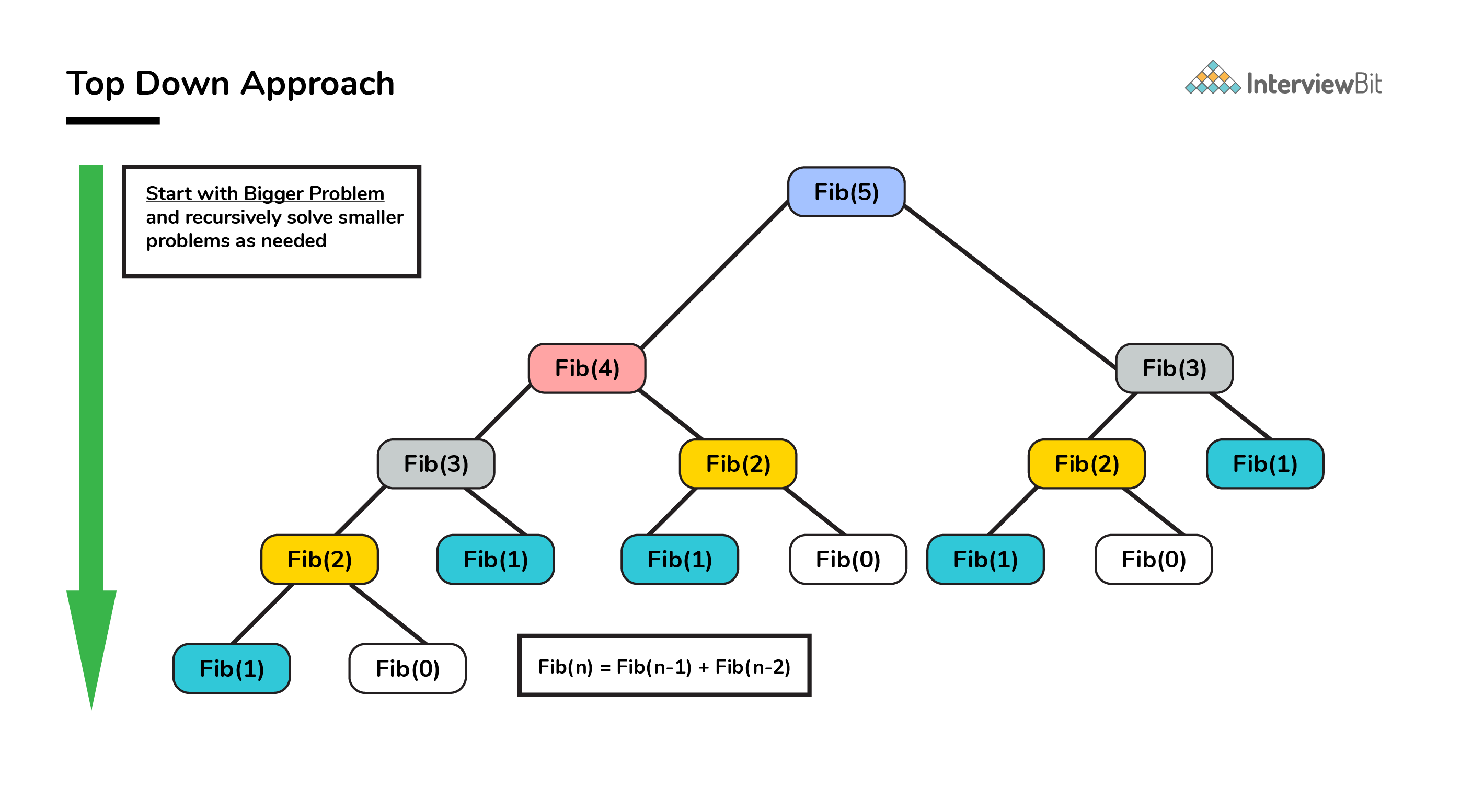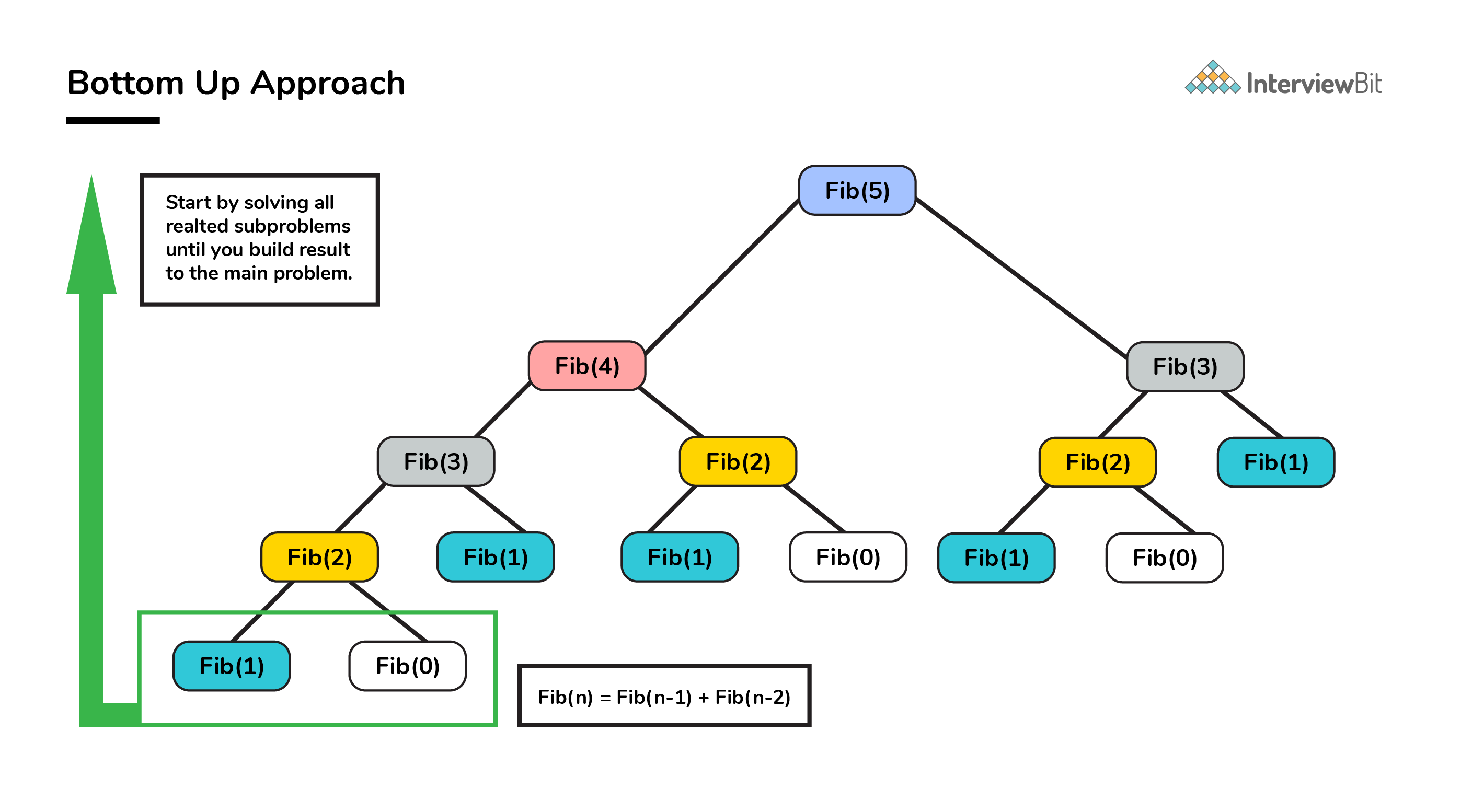Practice
Resources
Contests
Online IDE
New
Free Mock
Scaler
Practice
Improve your coding skills with our resources
Contests
Compete in popular contests with top coders
Scaler
Explore Offerings by SCALER## Welcome to Interviewbit, help us create the best experience for you!

Currently, You are a:College/University *
Enter the name of your college
Branch *
Year of completion *College/University *
Enter the name of your college
Branch *
Year of completion *Current Company *
Enter company name
Experience *## You're all set!Full name *
Email *

By creating an account, I acknowledge that I have read and agree to InterviewBit’s Terms and Privacy Policy .## Welcome back!

Email *Go to Problems

Be a Code Ninja!

# Dynamic Programming Methods

We shall continue with the example of finding the nth Fibonacci number in order to understand the DP methods available. We have the following two methods in DP technique. We can use any one of these techniques to solve a problem in optimised manner.

### Top Down Approach (Memoization)

• Top Down Approach is the method where we solve a bigger problem by recursively finding the solution to smaller sub-problems.• Whenever we solve a smaller subproblem, we remember (cache) its result so that we don’t solve it repeatedly if it’s called many times. Instead of solving repeatedly, we can just return the cached result.

• This method of remembering the solutions of already solved subproblems is called Memoization.

#### Pseudo Code and Analysis

Without Memoization

1. Think of a recursive approach to solving the problem. This part is simple. We already know `Fib(n) = Fib(n - 1) + Fib(n - 2)`.

2. Write a recursive code for the approach you just thought of.

``````/**
* Pseudo code for finding Fib(n) without memoization
* @Parameters: n : nth Fibonacci term needed
*
*/

int Fib(int n) {
if (n <= 1) return n;           //Fib(0)=0; Fib(1)=1
return Fib(n - 1) + Fib(n - 2);
}
``````
3. The time complexity of the above approach based on careful analysis on the property of recursion shows that it is essentially exponential in terms of n because some terms are evaluated again and again.

With Memoization

1. Save the results you get for every function run so that if `Fib(n)` is called again, you do not recompute the whole thing.
2. Instead of computing again and again, we save the value somewhere. This process of remembering the values of already run subproblem is called memoization.
3. Lets declare a global variable `memo` then.
``````    /**
* Pseudo code for finding Fib(n) with memoization
* @Parameters: n : nth Fibonacci term needed
*
*/
int memo = {0};
int Fib(int n) {
if (n <= 1) return n;

// If we have processed this function before,
// return the result from the last time.
if (memo[n] != 0) return memo[n];

// Otherwise calculate the result and remember it.
memo[n] = Fib(n - 1) + Fib(n - 2);
return memo[n];
}
``````
4. Let us now analyze the space and time complexity of this solution. We can try to improve this further if at all it is possible.
• Lets look at the space complexity first.
• We use an array of size n for remembering the results of subproblems. This contributes to a space complexity of `O(n)`.
• Since we are using recursion to solve this, we also end up using stack memory as part of recursion overhead which is also `O(n)`. So, overall space complexity is `O(n) + O(n) = 2 O(n) = O(n)`.
• Lets now look at the time complexity.
• Lets look at `Fib(n)`.

• When `Fib(n - 1)` is called, it makes a call to `Fib(n - 2)`. So when the call comes back to the original call from `Fib(n)``Fib(n-2)` would already be calculated. Hence the call to `Fib(n - 2)` will be O(1).

• Hence,

``````    T(n) = T(n - 1) + c where c is a constant.
= T(n - 2) + 2c
= T(n - 3) + 3c
= T(n - k) + kc
= T(0) + n * c = 1 + n * c = O(n)
``````

Thanks to Dynamic Programming, we have successfully reduced a exponential problem to a linear problem.

#### Implementation

``````/* C Program to find Nth Fibonacci Number using Memoization */

#include<stdio.h>

int Fibonacci_Series(int);
int memo = {0};
int main()
{
int N, FibN;

printf("\n Enter the Number to find Nth Fibonacci Number :  ");
scanf("%d", &N);

FibN = Fibonacci_Series(N);

printf("\n Fibonacci Number = %d", FibN);
return 0;
}

int Fibonacci_Series(int N)
{
if ( N == 0 )
return 0;
else if ( N == 1 )
return 1;

if (memo[n] != 0) return memo[n];
else{
memo[n]=Fibonacci_Series(N - 1) + Fibonacci_Series(N - 2)
return memo[n];
}
}``````
``````/* C++ Program to find Nth Fibonacci Number using Memoization */
#include <bits/stdc++.h>
using namespace std;
#define NIL -1

int memo;

/* Function to initialize NIL values in memo */
void initializeMemo()
{
int i;
for (i = 0; i < 100; i++)
memo[i] = NIL;
}

int fib(int n)
{
if (memo[n] == NIL)
{
if (n <= 1)
memo[n] = n;
else
memo[n] = fib(n - 1) + fib(n - 2);
}
return memo[n];
}

// Main Driver code
int main ()
{
int n = 10;
initializeMemo();
cout << "Fibonacci number : " << fib(n);
return 0;
}``````
``````/* Java Program to find Nth Fibonacci Number using Memoization */
public class Fibonacci
{
final int NIL = -1;

int memo[] = new int;

/* Function to initialize NIL values in memo */
void initializeMemo()
{
for (int i = 0; i < 100; i++)
memo[i] = NIL;
}

int fib(int n)
{
if (memo[n] == NIL)
{
if (n <= 1)
memo[n] = n;
else
memo[n] = fib(n-1) + fib(n-2);
}
return memo[n];
}

//Main Driver class
public static void main(String[] args)
{
Fibonacci fibonacci = new Fibonacci();
int n = 10;
fibonacci.initializeMemo();
System.out.println("Fibonacci number : " + fibonacci.fib(n));
}

}``````
``````# Python Program to find Nth Fibonacci Number using Memoization
def fib(n, memo):
# Base case
if n == 0 or n == 1 :
memo[n] = n

# If memo[n] is not evaluated before then calculate it
if memo[n] is None:
memo[n] = fib(n-1 , memo)  + fib(n-2 , memo)

# return the value corresponding value of n
return memo[n]

# Driver program
def main():
n = 10
# Declaration of memo
memo = [None]*(101)

# Calculate and display result
print("Fibonacci Number is " + str(fib(n, lookup)))

if __name__=="__main__":
main() ``````

### Bottom Up Approach (Tabulation)

• As the name indicates, bottom up is the opposite of the top-down approach which avoids recursion.

• Here, we solve the problem “bottom-up” way i.e. by solving all the related subproblems first. This is typically done by populating into an n-dimensional table.• Depending on the results in the table, the solution to the original problem is then computed. This approach is therefore called as “Tabulation”.

#### Pseudo Code and Analysis

1. We already know `Fib(n) = Fib(n - 1) + Fib(n - 2)`.
2. Based on the above relation, we calculate the results of smaller subproblems first and then build the table.
``````/**
* Pseudo code for finding Fib(n) using tabulation
* @Parameters: n : nth Fibonacci term needed
* local variable dp[] table built to store results of smaller subproblems
*/

int Fib(int n) {
if (n==0) return 0;
int dp[] = new int[n+1];

//define base cases
dp = 0;
dp = 1;

//Iteratively compute the results and store
for(int i=2; i<=n; i++)
dp[i] = dp[i-1] + dp[i-2];

//return the value corresponding to nth term
return dp[n];
}
``````
1. Analyze the space and time requirements
• Lets look at the space complexity first.
• In this case too, we use an array of size n for remembering the results which contributes to a space complexity of `O(n)`.
• We can further reduce the space complexity from `O(N)` to `O(1)` by just using 2 variables. This is left as an assignment to the reader.
• Lets now look at the time complexity.
• Lets look at `Fib(n)`.
• Here, we solve each subproblem only once in iterative manner. So the time complexity of the algorithm is also O(N).
• Again thanks to DP, we arrived at solution in linear time complexity.

#### Implementation

``````/* C Program to find Nth Fibonacci Number using Tabulation */

#include<stdio.h>
int fib(int n)
{
int dp[n+1];
int i;
//base cases
dp = 0;   dp = 1;

//calculating and storing the values
for (i = 2; i <= n; i++)
dp[i] = dp[i-1] + dp[i-2];

return dp[n];
}

int main ()
{
int n = 10;
printf("Fibonacci number : %d ", fib(n));
return 0;
}``````
``````/* C++ Program to find Nth Fibonacci Number using Tabulation */
#include <bits/stdc++.h>
using namespace std;

int fib(int n)
{
int dp[n+1];
int i;

//base cases
dp = 0;   dp = 1;

//calculating and storing the values
for (i = 2; i <= n; i++)
dp[i] = dp[i-1] + dp[i-2];

return dp[n];
}

// Main Driver code
int main ()
{
int n = 10;
cout << "Fibonacci number : " << fib(n);
return 0;
}  ``````
``````/* Java Program to find Nth Fibonacci Number using Tabulation */
public class Fibonacci
{
int fib(int n){
int dp[] = new int[n+1];
//base cases
dp = 0;
dp = 1;

//calculating and storing the values
for (int i = 2; i <= n; i++)
dp[i] = dp[i-1] + dp[i-2];
return dp[n];
}

public static void main(String[] args)
{
Fibonacci fibonacci = new Fibonacci();
int n = 10;
System.out.println("Fibonacci number : " + fibonacci.fib(n));
}

} ``````
``````# Python Program to find Nth Fibonacci Number using Tabulation
def fib(n):

# dp array declaration
dp = *(n+1)

# base case
dp = 1

# calculating and storing the values
for i in xrange(2 , n+1):
dp[i] = dp[i-1] + dp[i-2]
return dp[n]

# Driver program
def main():
n = 10
print("Fibonacci number : " + str(fib(n)))

if __name__=="__main__":
main() ``````

## Serious about Learning Programming ?

Learn this and a lot more with Scaler Academy's industry vetted curriculum which covers Data Structures & Algorithms in depth.

## Dynamic Programming Problems

2d string dp
Greedy or dp
Problem Score Companies Time Status
Tushar's Birthday Bombs 200
78:13
Jump Game Array 225 41:16
Min Jumps Array 300 71:44
Tree dp
Problem Score Companies Time Status
Max edge queries! 200 56:34
Max Sum Path in Binary Tree 400 55:07
Suffix / prefix dp
Problem Score Companies Time Status
Sub Matrices with sum Zero 200
73:24
Coin Sum Infinite 225 64:26
Max Product Subarray 300 65:03
Best Time to Buy and Sell Stocks I 300 28:13
Arrange II 350 71:34
Derived dp
Problem Score Companies Time Status
Chain of Pairs 200 42:09
Max Sum Without Adjacent Elements 225 58:10
Merge elements 300 57:43
Knapsack
Problem Score Companies Time Status
Flip Array 200
78:42
Tushar's Birthday Party 200 70:50
0-1 Knapsack 200 47:57
Equal Average Partition 350 71:49
Problem Score Companies Time Status
Best Time to Buy and Sell Stocks II 225 40:18
Dp optimized backtrack
Problem Score Companies Time Status
Word Break II 350 67:29
Multiply dp
Problem Score Companies Time Status
Unique Binary Search Trees II 400 36:06
Count Permutations of BST 400
62:18
Breaking words
Problem Score Companies Time Status
Palindrome Partitioning II 400 62:02
Word Break 400 67:00

Problem Score Companies Time Status
Potions 200 56:48
Dice Throw 400 49:19
Double Increasing Series 200 45:07
Dice Rolls 300 27:36
Palindromic Substrings 200 25:37Free Mock Assessment
Help us know you better for the best experience
Current Employer *
Enter company name